# Shortest Common Supersequence | (DP – 31)

Problem Statement: Shortest Common Supersequence

We are given two strings ‘S1’ and ‘S2’. We need to return their shortest common supersequence. A supersequence is defined as the string which contains both the strings S1 and S2 as subsequences.

```Example: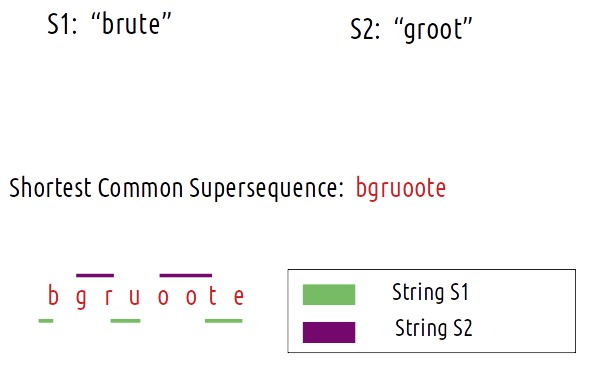```

We need to return the length of the longest common subsequence and the string as well.

Problem Link: Shortest Supersequence

### Intuition:

If we keep the “shortest” criteria aside, what can be a way to generate a supersequence given two strings. One easy way is to concat the given strings (write one after the other), this will always give us a supersequence for any pair of given strings.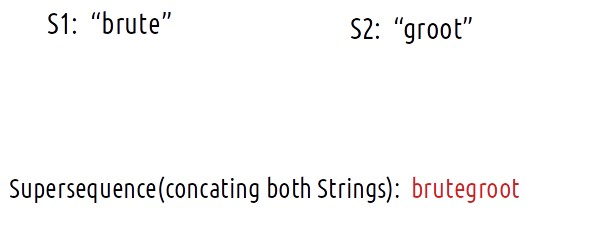This can be said as the worst case with time complexity of O(n+m), where n and are the lengths of strings S1 and S2 respectively.

How can we improve from this naive approach?

If we think a little, there are some common characters that we can avoid writing for both the strings separately. These common characters can’t be all the common characters. They are the characters that are common and come in the same order. In other words, they are the characters of the longest common subsequence

In an optimum solution, the characters of the longest common subsequence are written only once and other characters are placed around them. For every character that belongs to the longest common subsequence, the non-lcs characters coming before them in the strings S1 and S2 are placed before the lcs-character in the answer string. The below figure explains this: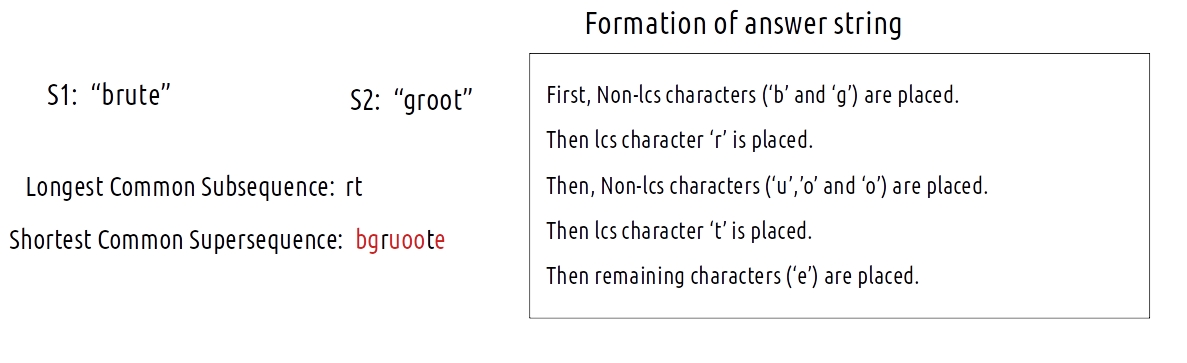### Length of Shortest Common Supersequence?

From the explanation above, we can see that characters of lcs need to be covered only once. Therefore, the length of the shortest Common supersequence = n + m -k, where (n and m are lengths of S1 and S2, and k is the length of the lcs string).

Finding the supersequence string

Now, instead of the length, we are interested in finding the shortest supersequence string itself. Readers are highly advised to read the article Print Longest Common Subsequence.

### Intuition:

When we form the DP table to calculate the longest common subsequence (as done in Print Longest Common Subsequence) we have all the information of characters that are coming in the lcs string and characters that don’t. We use this same DP table to form the shortest common supersequence.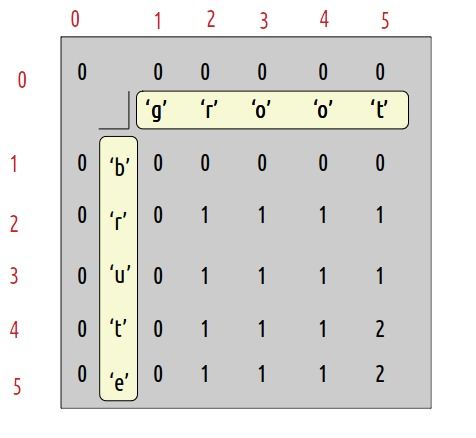To frame the string, we need to understand how the dp table was formed and work in the reverse process.

Now, let us see what were the conditions that we used while forming the dp array:

• if(S1[i-1] == S2[j-1]), then return 1 + dp[i-1][j-1]
• if(S1[i-1] != S2[j-1]) , then return 0 + max(dp[i-1][j],dp[i][j-1])

Approach:

We will start from the right-most cell of the dp array, initially i=n and j=m

To form the string, we will work in a reverse manner.

• if(S1[i-1] == S2[j-1]), this means the character is an lcs character and needs to be included only once from both the strings, so we add it to the ans string and reduce both i and j by 1. We reduce them simultaneously to make sure the character is counted only once.
• if(S1[i-1] != S2[j-1]), this means that the character is a non-lcs character and then we move the pointer to the top cell or left cell depending on which is greater. This way non-lcs characters will be included separately in the right order.

The algorithm steps are stated below:

• We start from cell dp[n][m]. Initially i=n and j=m.
• At every cell, we will check if S1[i-1] == S2[j-1], if it is then it means this character is a part of the longest common subsequence. So we will push it to the ans string str. Then we will move to the diagonally top-left(↖)  cell by assigning i to i-1 and j to j-1.
• Else, this character is not a part of the longest common subsequence so we include it in ans string. Originally this cell got its value from its left cell (←) or from its top cell (↑). Whichever cell’s value will be more of the two, we will move to that cell.
• We will continue till i>0 and j>0, failing it we will break from the loop.
• After breaking, either i>0 or j>0 (only one condition will fail to break from the while loop), if(i>0) we push all the characters from S1 to ans string, else if(j>0), we push all the remaining characters from S2.
• At last, we reverse the ‘ans’ string and we get our answer.

### Dry Run:

At starting i=5 and j=5.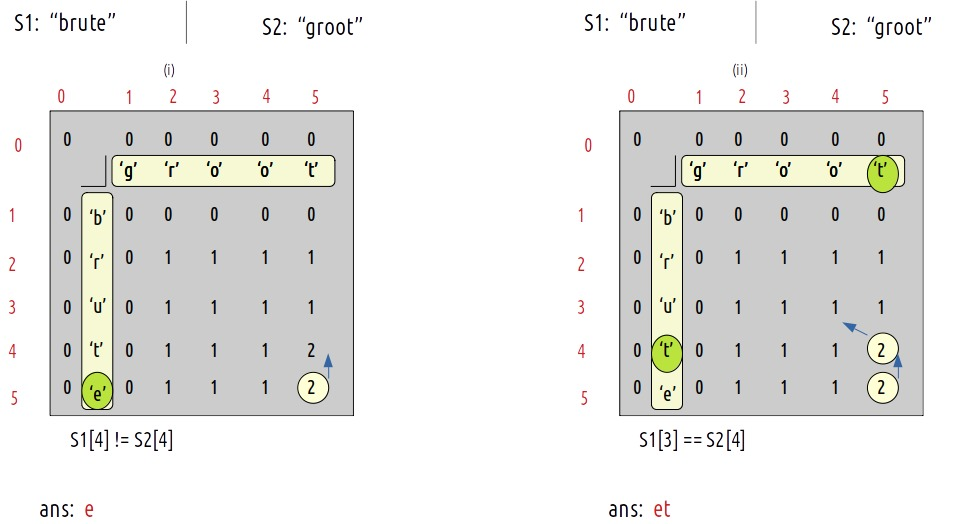(i) As S1 != S2, we move to the top cell(↑) as its value is greater than the left cell(←)  but before moving as we are leaving row 5(i=5) and will not return to it so we add S1 to our ans string.

(ii) As S1 == S2, this is an lcs-character. We add the current character to the ans string(and move to i-1 and j-1 cell) i.e top-left(↖). Reducing i and j simultaneously helps in adding the lcs character only once in the ans string.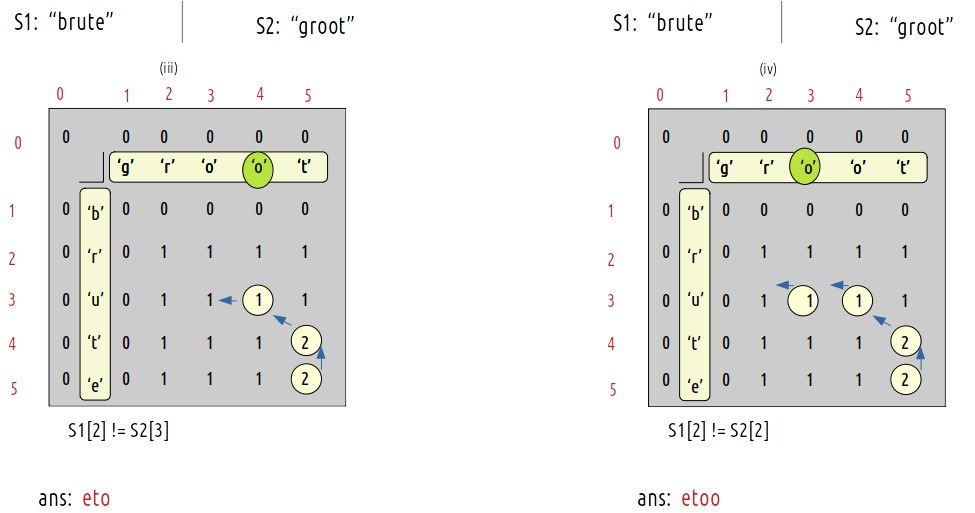(iii) As S1 != S2, we move to the left cell(←) as its value is greater than or equal to the top cell(↑) but before moving as we are leaving column 4 (j=4) and will not return to it so we add S2 to our ans string.

(iv) As S1 != S2, we move to the left cell(←) as its value is greater than or equal to the top cell(↑) but before moving as we are leaving column 3 (j=3) and will not return to it so we add S2 to our ans string.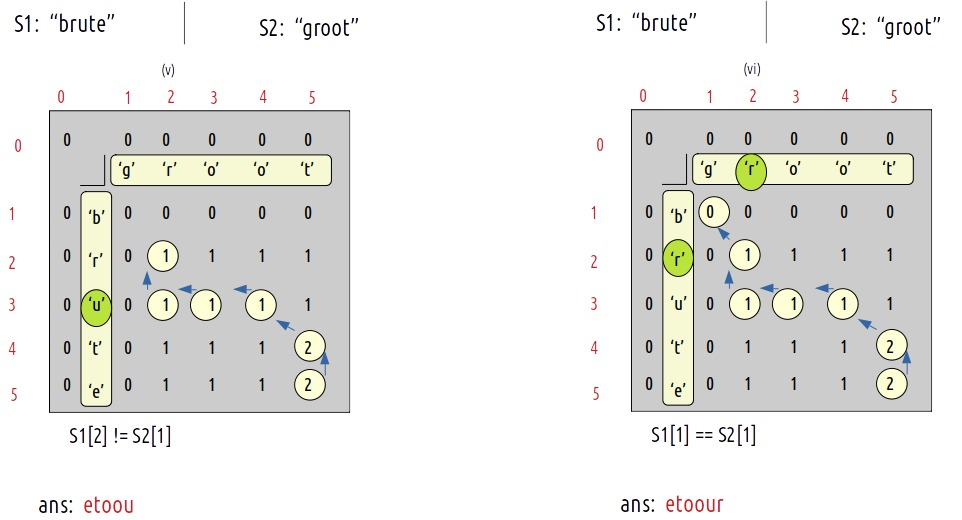(v) As S1 != S2, we move to the top cell(↑) as its value is greater than the left cell(←)  but before moving as we are leaving row 3(i=3) and will not return to it so we add S1 to our ans string.

(vi) As S1 == S2, this is an lcs-character. We add the current character to the ans string(and move to i-1 and j-1 cell) i.e top-left(↖).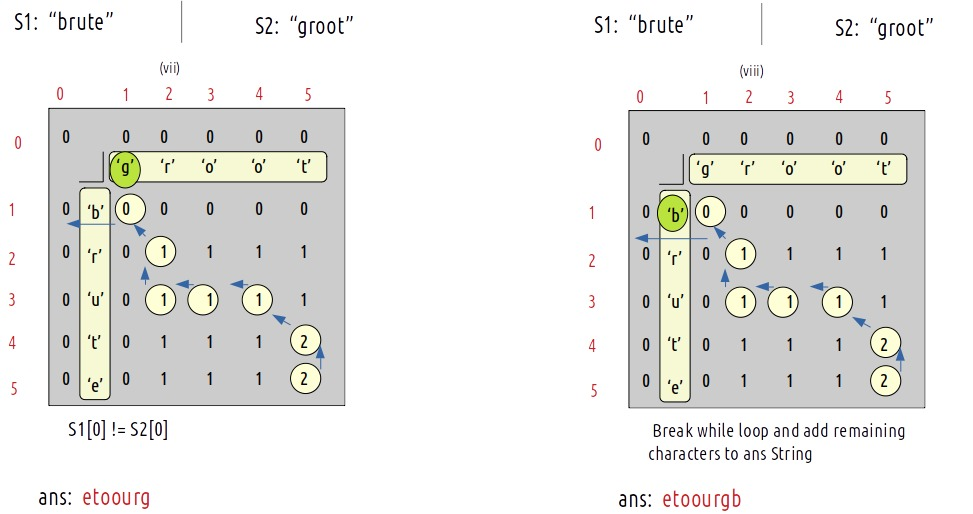(vii)  As S1 != S2, we move to the left cell(←) as its value is greater than or equal to the top cell(↑) but before moving as we are leaving column 1 (j=1) and will not return to it so we add S2 to our ans string.

(viii) As j=0, we have reached the exit condition of the while loop, so we will break from it but still there are some characters left in the other string. We will simply add them in the ans string.

(ix) At last, we will return the reverse of the ans string as the final answer.

Code:

## C++ Code

``````#include <bits/stdc++.h>

using namespace std;

string shortestSupersequence(string s1, string s2){

int n = s1.size();
int m = s2.size();

vector < vector < int >> dp(n + 1, vector < int > (m + 1, 0));
for (int i = 0; i <= n; i++) {
dp[i] = 0;
}
for (int i = 0; i <= m; i++) {
dp[i] = 0;
}

for (int ind1 = 1; ind1 <= n; ind1++) {
for (int ind2 = 1; ind2 <= m; ind2++) {
if (s1[ind1 - 1] == s2[ind2 - 1])
dp[ind1][ind2] = 1 + dp[ind1 - 1][ind2 - 1];
else
dp[ind1][ind2] = 0 + max(dp[ind1 - 1][ind2], dp[ind1][ind2 - 1]);
}
}

int len = dp[n][m];
int i = n;
int j = m;

int index = len - 1;
string ans = "";

while (i > 0 && j > 0) {
if (s1[i - 1] == s2[j - 1]) {
ans += s1[i-1];
index--;
i--;
j--;
} else if (dp[i - 1][j] > dp[i][j - 1]) {
ans += s1[i-1];
i--;
} else {
ans += s2[j-1];
j--;
}
}

//Adding Remaing Characters - Only one of the below two while loops will run

while(i>0){
ans += s1[i-1];
i--;
}
while(j>0){
ans += s2[j-1];
j--;
}

reverse(ans.begin(),ans.end());

return ans;
}

int main() {

string s1 = "brute";
string s2 = "groot";

cout << "The Longest Common Supersequence is "<<shortestSupersequence(s1,s2);
}
``````

Output:

The Longest Common Supersequence is bgruoote

Time Complexity: O(N*M)

Reason: There are two nested loops

Space Complexity: O(N*M)

Reason: We are using an external array of size (N*M).

## Java Code

``````import java.util.*;

class TUF{
static String shortestSupersequence(String s1, String s2){

int n = s1.length();
int m = s2.length();

int[][] dp =new int[n+1][m+1];
for (int i = 0; i <= n; i++) {
dp[i] = 0;
}
for (int i = 0; i <= m; i++) {
dp[i] = 0;
}

for (int ind1 = 1; ind1 <= n; ind1++) {
for (int ind2 = 1; ind2 <= m; ind2++) {
if (s1.charAt(ind1 - 1) == s2.charAt(ind2 - 1))
dp[ind1][ind2] = 1 + dp[ind1 - 1][ind2 - 1];
else
dp[ind1][ind2] = 0 + Math.max(dp[ind1 - 1][ind2], dp[ind1][ind2 - 1]);
}
}

int len = dp[n][m];
int i = n;
int j = m;

int index = len - 1;
String ans = "";

while (i > 0 && j > 0) {
if (s1.charAt(i - 1) == s2.charAt(j - 1)) {
ans += s1.charAt(i-1);
index--;
i--;
j--;
} else if (dp[i - 1][j] > dp[i][j - 1]) {
ans += s1.charAt(i-1);
i--;
} else {
ans += s2.charAt(j-1);
j--;
}
}

//Adding Remaing Characters - Only one of the below two while loops will run

while(i>0){
ans += s1.charAt(i-1);
i--;
}
while(j>0){
ans += s2.charAt(j-1);
j--;
}

String ans2=new StringBuilder(ans).reverse().toString();

return ans2;
}

public static void main(String args[]) {

String s1 = "brute";
String s2 = "groot";

System.out.println("The Longest Common Supersequence is "+shortestSupersequence(s1,s2));
}
}``````

Output:

The Longest Common Supersequence is bgruoote

Time Complexity: O(N*M)

Reason: There are two nested loops

Space Complexity: O(N*M)

Reason: We are using an external array of size (N*M).

Special thanks to Anshuman Sharma for contributing to this article on takeUforward. If you also wish to share your knowledge with the takeUforward fam, please check out this article